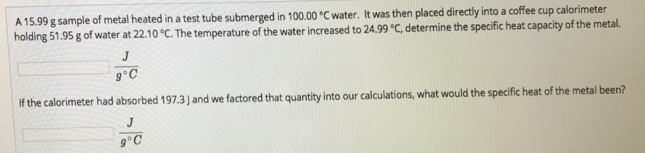Chemistry Calorimetry Solution: A 15.99 g sample of metal heated in a test tube su...

# Solution: A 15.99 g sample of metal heated in a test tube submerged in 100.00°C water. It was then placed directly into a coffee cup calorimeter holding 51.95 g of water at 22.10°C. The temperature of the water increased to 24.99°C, determine the specific heat capacity of the metal. If the calorimete had absorbed 197.3 J and we factored that quantity into our calculation, what would the specific heat of the metal been?

Problem

A 15.99 g sample of metal heated in a test tube submerged in 100.00°C water. It was then placed directly into a coffee cup calorimeter holding 51.95 g of water at 22.10°C. The temperature of the water increased to 24.99°C, determine the specific heat capacity of the metal.

If the calorimete had absorbed 197.3 J and we factored that quantity into our calculation, what would the specific heat of the metal been?View Complete Written Solution